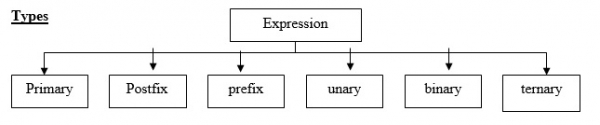# Explain different types of expressions in C program

CServer Side ProgrammingProgramming

An expression is a combination of operators and operands which reduces to a single value. An operation is performed on a data item which is called an operand. An operator indicates an operation to be performed on data.

For example, z = 3+2*1

z = 5• Primary expressions − It is an operand which can be a name, a constant or any parenthesized expression. Example − c = a+ (5*b);

• Postfix expressions − In a postfix expression, the operator will be after the operand. Example − ab+

• Prefix expressions − n a prefix expression, the operator is before the operand. Example − +ab

• Unary expression − It contains one operator and one operand. Example − a++, --b

• Binary expression − t contains two operands and one operator. Example − a+b, c-d

• Ternary expression − It contains three operands and one operator. For Example, Exp1? Exp2 − Exp3. If Exp1 is true, Exp2 is executed. Otherwise, Exp3 is executed.

## Example

Given below is the C program explaining the different types of expressions in C language −

Live Demo

#include<stdio.h>
int main(){
int a,b,c,d,z;
int p,q,r,s,t,u,v;
printf("enter the values of a,b,c,d:\n");
scanf("%d%d%d%d",&a,&b,&c,&d);
r=a++;
s=--b;
t=a+b;
u=c-d;
v=a+(5*b);
z = (5>3) ? 1:0;
printf("unaryexpression=%d\nunary expression=%d\n Binary
expression=%d\nBinary expression=%d\nPrimary expression=%d\nTernary expression=%d\n",r,s,t,u,v,z);
}

## Output

You will see the following output −

enter the values of a,b,c,d:
2 3 4 6
unary expression=2
unary expression=2
Binary expression=5
Binary expression=-2
Primary expression=13
Ternary expression=1